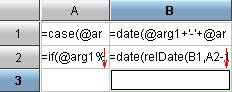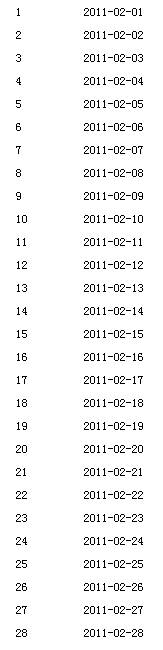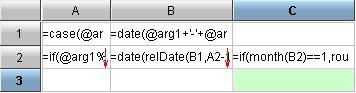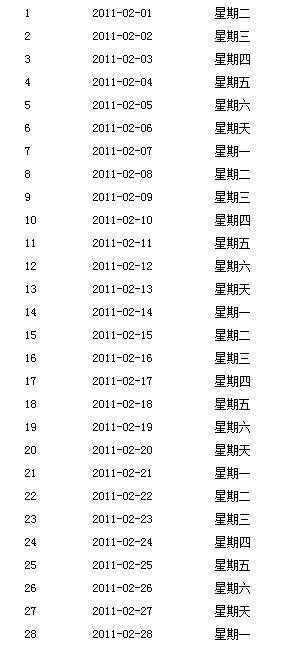# 润乾 -- 判断任意日期的星期几A1单元格根据参数年份和参数月份判断该月的天数；

A2单元格根据参数年份判断是否是润年，如果是则对二月份进行判断计算出该月总天数；

B1单元格为年份和月份拼成的日期类型，

B2单元格根据B1单元格拼出的该月第一天用relDate函数通过A2单元格获取的该月总共的天数去计算该月最后一天；W= (d+2*m+3*(m+1)/5+y+y/4-y/100+y/400) mod 7

if(month(B2)==1,round((day(B2)+(2*(month(B2)+12))+(3*((month(B2)+12)+1)/5)+(year(B2)-1)+((year(B2)-1)/4)-((year(B2)-1)/100)+((year(B2)-1)/400))%7,0),month(B2)==2,(round((day(B2)+(2*(month(B2)+12))+(3*((month(B2)+12)+1)/5)+(year(B2)-1)+((year(B2)-1)/4)-((year(B2)-1)/100)+((year(B2)-1)/400))%7,0))+1.0,round((day(B2)+(2*month(B2))+(3*(month(B2)+1)/5)+year(B2)+(year(B2)/4)-(year(B2)/100)+(year(B2)/400))%7,0))

if(map(list(1,2,3,4,5,6,7),list(“星期一“,”星期二“,”星期三“,”星期四“,”星期五“,”星期六“,”星期天“)))即可实现如下图所示效果: